# Insert 3

Insert five arithmetic progression members between -7 and 3/2.

a2 =  -5.5833
a3 =  -4.1667
a4 =  -2.75
a5 =  -1.3333
a6 =  0.0833

### Step-by-step explanation:

${a}_{3}={a}_{2}+d=\left(-5.5833\right)+1.4167=-4.1667$
${a}_{4}={a}_{3}+d=\left(-4.1667\right)+1.4167=-2.75$
${a}_{5}={a}_{4}+d=\left(-2.75\right)+1.4167=-1.3333$
${a}_{6}={a}_{5}+d=\left(-1.3333\right)+1.4167=0.0833$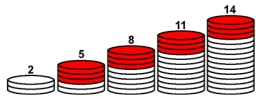Did you find an error or inaccuracy? Feel free to write us. Thank you!## Related math problems and questions:

• Harmonic seriesInsert four members between 5/3 and 5/11 to form harmonic series (means).
• Insert 7Insert five harmonic means between 3 and 18
• AP members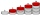What is the value of x2, x3, x4, x5…of the terms (of arithmetic progression) when x1 = 8 and x6 = 20?
• Negative differenceFind four arithmetic progression members between 7 and -6.
• Two geometric progressions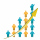Insert several numbers between numbers 6 and 384 so that they form with the given GP numbers and that the following applies: a) the sum of all numbers is 510 And for another GP to apply: b) the sum of entered numbers is -132 (These are two different geome
• GP membersThe geometric sequence has 10 members. The last two members are 2 and -1. Which member is -1/16?
• Insert into GPBetween numbers 5 and 640, insert as many numbers to form geometric progression so the sum of the numbers you entered will be 630. How many numbers must you insert?
• SequenceBetween numbers 1 and 53 insert n members of the arithmetic sequence that its sum is 702.
• Difference AP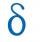Calculate the difference of arithmetic progression if the sum of its first 19 members Sn = 8075 and the first member is a1 = 20
• Fifth memberDetermine the fifth member of the arithmetic progression, if the sum of the second and fifth members equal to 73, and difference d = 7.
• Geometric progressionIn geometric progression, a1 = 7, q = 5. Find the condition for n to sum first n members is sn≤217.
• GP - three membersThe second and third of a geometric progression are 24 and 12(c+1), respectively, given that the sum of the first three terms of progression is 76. determine the value of c.
• Insert 6Insert four harmonic means between 3/7 and 3/19
• Insert 4Insert three arithmetic means between 3 and 63.
• Right-angled triangle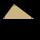Determine the content of a right triangle whose side lengths form successive members of an arithmetic progression and the radius of the circle described by the triangle is 5 cm.
• Insert 5Insert five harmonic means between 1/2 and 1/26
• Five element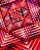The geometric sequence is given by quotient q = 1/2 and the sum of the first six members S6 = 63. Find the fifth element a5.